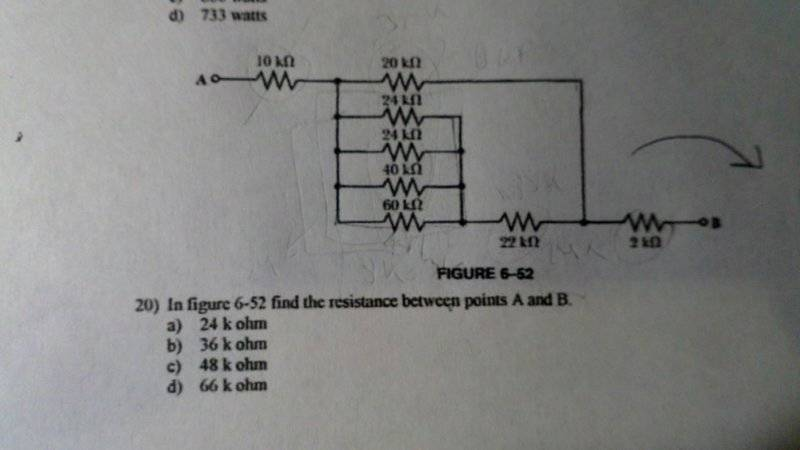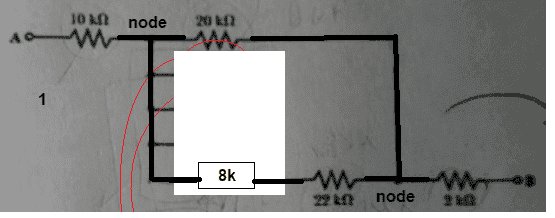# Combination Circuit Help (pic)

• atoms

#### atoms

Member advised to use the formatting template for all homework help requests
Could somebody help me out with this combination circuit? The answer that I get is 62K but that is not one of the answers. At first, I thought the 10kohm and 20kohm wherein series and the other side as well. Then I thought they are all in parallel because of the different current flow they all can take but I couldn't figure out the answer. Any help will be appreciated.#### Attachments

So show how you did it.

I did the parallel resistors to get a single value.
Then the one in parallel.
Then added the two series resistances.

They've made the values all work out easily. There are no difficult sums. You could probably do it in your head, but better write it down for checking.

So show how you did it.

I did the parallel resistors to get a single value.
Then the one in parallel.
Then added the two series resistances.

They've made the values all work out easily. There are no difficult sums. You could probably do it in your head, but better write it down for checking.

So from what you are saying is getting the 24k,24k,40k,and 60k in parallel getting 8k then adding the 22k and 2k which would be 32k. Finding the parallel of the 32k with the 20k and getting 12k, adding the 10k which would be 22 and still not getting the right answer.

So from what you are saying is getting the 24k,24k,40k,and 60k in parallel getting 8k Yes
then adding the 22k and 2k which would be 30k. No. First, 8+22+2 = 32. But you can't add all three yet. So keep one for later.
Finding the parallel of the 30k with the 20k and getting 12k, Yes
adding the 10k which would be 22 Yes
and still not getting the right answer. Only 3 out of 4 yesesBTW. You haven't shown how you got 62k yet

#### Attachments

Last edited:
View attachment 232555
BTW. You haven't shown how you got 62k yet
So the outer resisitors I add at the end once I calculate the inside. Thank you

I added the 32 from the bottom and the 30 on top which was terribly wrong

yes thank you